# Wilcox Solar Observatory Magnetograms

## #8080 - 8089, 2004.09.02 - 2004.09.09```WSO Magnetogram sn=8080	 observed at 2004:09:02_21h:37m:51s
Central Meridian Carrington Longitude CT2020:158
Sky = 0 (5 worst)
trouble=00004000 NOTE:
12 intensity variance exceeds 5.0
```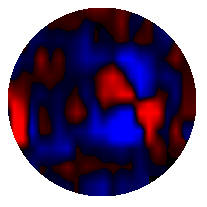```WSO Magnetogram sn=8081	 observed at 2004:09:02_23h:45m:37s
Central Meridian Carrington Longitude CT2020:156
Sky = 0 (5 worst)
trouble=00004000 NOTE:
12 intensity variance exceeds 5.0
```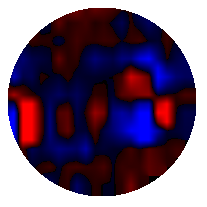```WSO Magnetogram sn=8082	 observed at 2004:09:03_22h:42m:42s
Central Meridian Carrington Longitude CT2020:144
Sky = 0 (5 worst)
trouble=00004000 NOTE:
12 intensity variance exceeds 5.0
```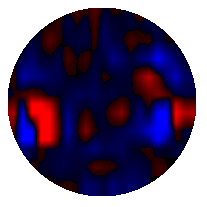```WSO Magnetogram sn=8083	 observed at 2004:09:04_22h:15m:07s
Central Meridian Carrington Longitude CT2020:131
Sky = 0 (5 worst)
trouble=00004000 NOTE:
12 intensity variance exceeds 5.0
```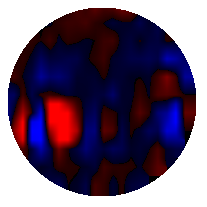```WSO Magnetogram sn=8084	 observed at 2004:09:05_21h:44m:10s
Central Meridian Carrington Longitude CT2020:118
Sky = 0 (5 worst)
trouble=00004000 NOTE:
12 intensity variance exceeds 5.0
```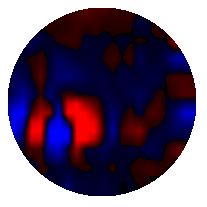```WSO Magnetogram sn=8085	 observed at 2004:09:06_22h:52m:13s
Central Meridian Carrington Longitude CT2020:104
Sky = 0 (5 worst)
trouble=00004000 NOTE:
12 intensity variance exceeds 5.0
```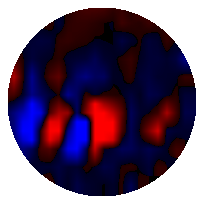```WSO Magnetogram sn=8086	 observed at 2004:09:07_21h:17m:09s
Central Meridian Carrington Longitude CT2020:092
Sky = 0 (5 worst)
trouble=00004000 NOTE:
12 intensity variance exceeds 5.0
```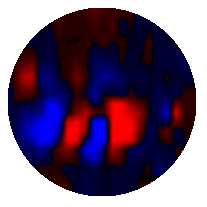```WSO Magnetogram sn=8087	 observed at 2004:09:08_22h:27m:11s
Central Meridian Carrington Longitude CT2020:078
Sky = 0 (5 worst)
trouble=00004000 NOTE:
12 intensity variance exceeds 5.0
```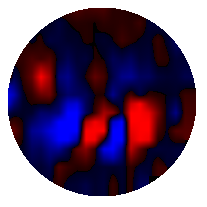```WSO Magnetogram sn=8088	 observed at 2004:09:09_21h:18m:54s
Central Meridian Carrington Longitude CT2020:065
Sky = 0 (5 worst)
trouble=00004000 NOTE:
12 intensity variance exceeds 5.0
``````WSO Magnetogram sn=8089	 observed at 2004:09:09_23h:26m:26s
Central Meridian Carrington Longitude CT2020:064
Sky = 0 (5 worst)
trouble=00004000 NOTE:
12 intensity variance exceeds 5.0
```